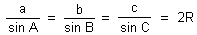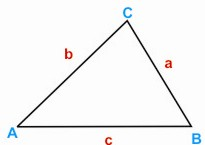HOME MATH DICTIONARY DOWNLOAD FEEDBACK DISCLAIMER
 Question: What is Sine Rule ? Answer: In any triangle if a, b and c are the lengths of sides of a triangle and A, B, C are the angles in triangle with A opposite to a etc then, the rule of sine states :This rule is useful when computing the remaining sides of a triangle if two angles and a side are known, a common problem in the technique of triangulation.